### Home > CALC > Chapter 4 > Lesson 4.3.1 > Problem4-100

4-100.
1.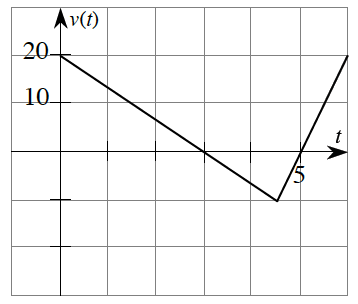The graph at right shows the velocity (in miles per hour) of a car during a road trip. At time t = 0, the car was on the Golden Gate Bridge heading north. Homework Help ✎

1. Find a function for v(t).

2. How far north has the car traveled at 3 hours? At 4 hours?

3. Explain what happened to the car between 3 ≤ t ≤ 5 hours.

4. Set up an integral to represent the displacement from 0 ≤ t ≤ 6.

5. Set up an integral to represent the total distance from 0 ≤ t ≤ 6.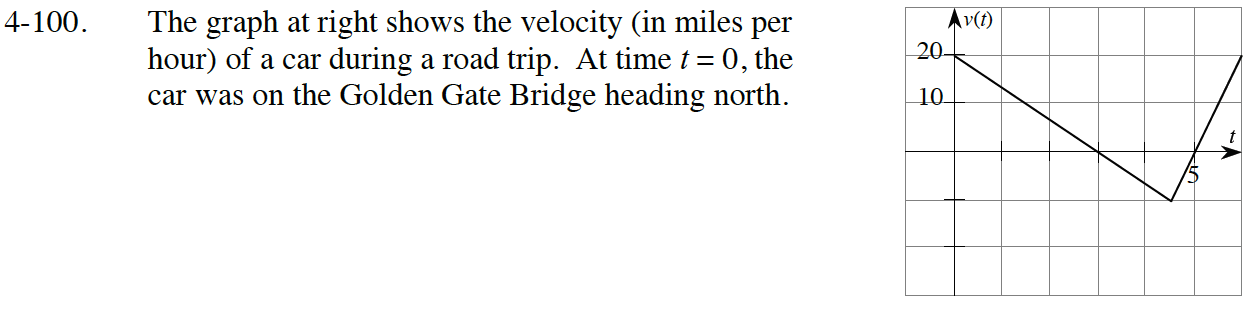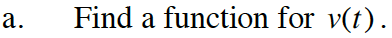Write an equation for each line segment.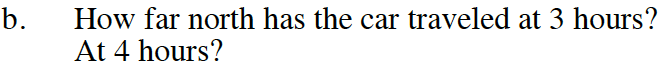Since this is a velocity graph, calculate the area under the curve for the given interval.

$\int_{0}^{3}v(t)dt\text{ and }\int_{0}^{4}v(t)dt=\int_{0}^{2}v(t)dt$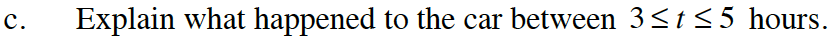For 3 ≤ t ≤ 5, the function values are negative. This is a velocity graph, so what does negative velocity tell you about position?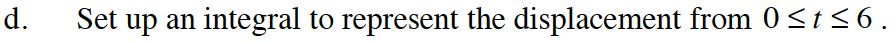$\text{displacement }=\int_{a}^{b}\text{velocity } dt$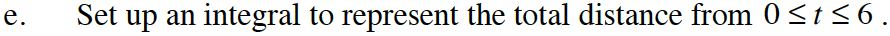$\text{total distance }=\int_{a}^{b}\text{speed } dt=\int_{a}^{b}\left | \text{velocity} \right |dt$